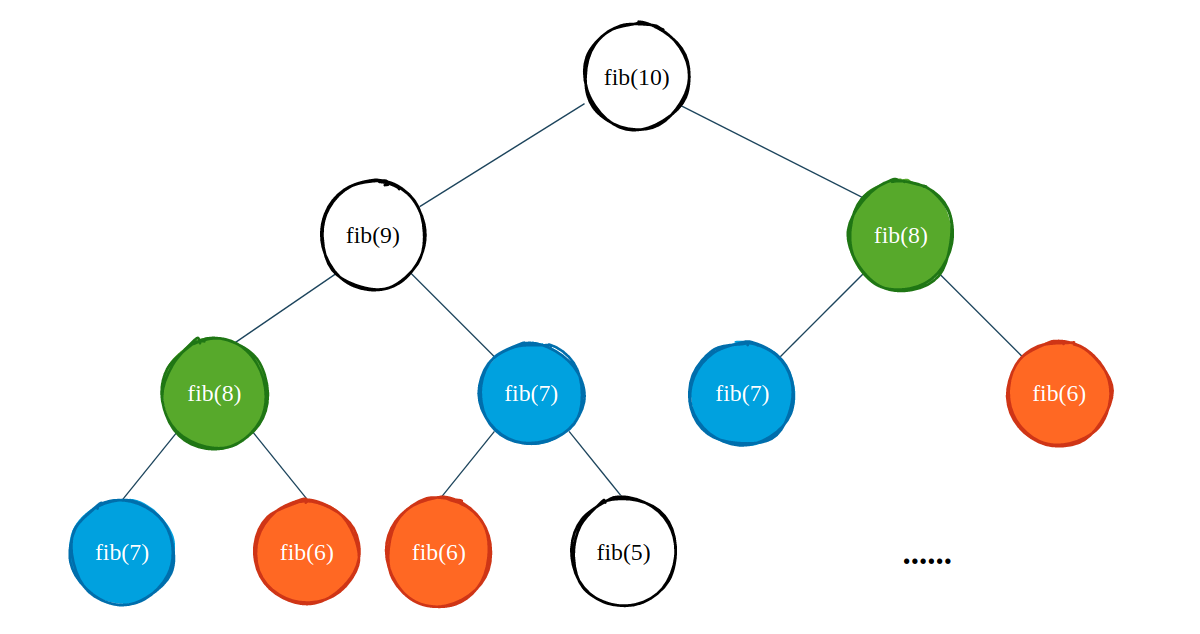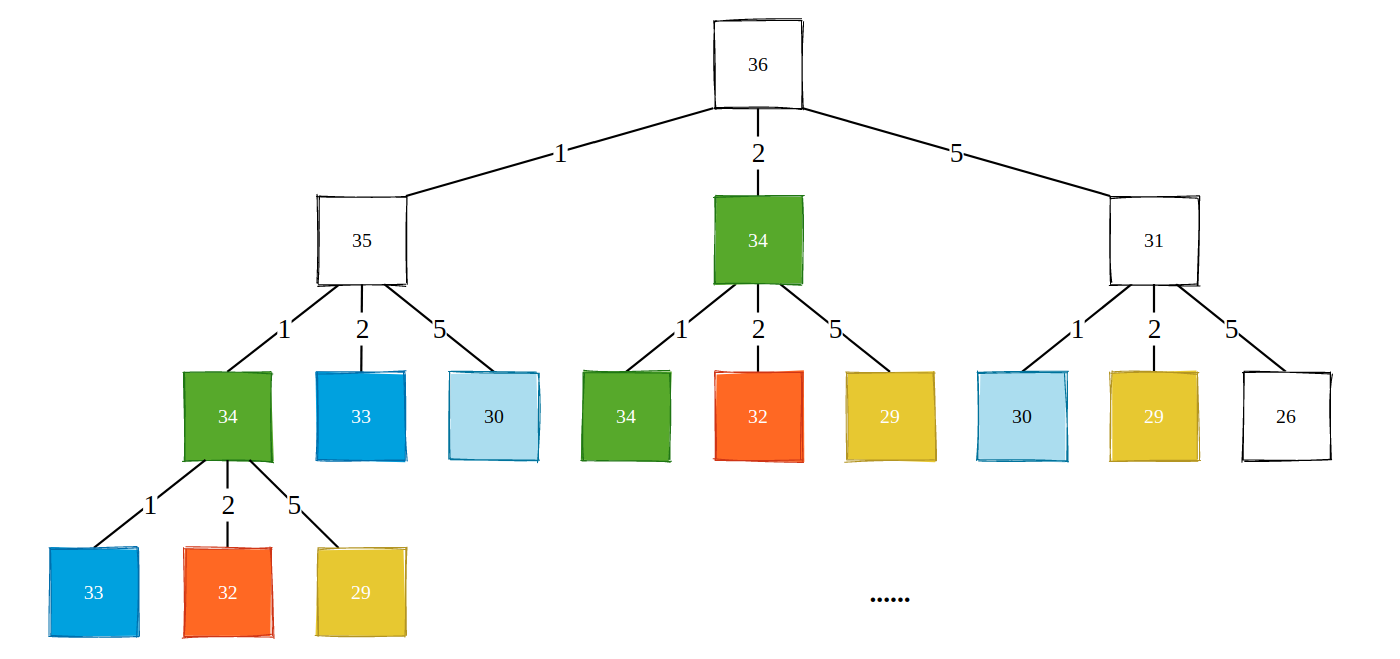### 1. 从斐波那契数列中找到 DP 模板

``````// 计算第 n 个斐波那契数
int fib(int n) {
if (n <= 2) return 1;
return fib(n-1) + fib(n-2);
}
``````

99% 的程序员闭着眼睛都能写出上面的递归程序，但是如果我们运行这个程序的话，就会发现当 `n` 等于 50 的时候，程序需要花费几十秒的时间才能输出结果（有没有兄弟用 Apple M1 试下…）。为什么需要这么长的时间?我们以 `fib(10)` 为例，画出递归树:``````int fibUseMemory(int n, vector<int> &memory) {
if (n <= 2) return 1;
if (memory[n]) return memory[n];
int res = fibUseMemory(n-1, memory) + fibUseMemory(n-2, memory);
memory[n] = res;
return res;
}
``````

``````int fibUseDP(int n) {
vector<int> memory = vector<int>(n+1, 0);
memory = 1, memory = 1;       // 边界情况处理
for (int i = 2; i <= n; i++)        // 递推过程
memory[i] = memory[i-1] + memory[i-2];
return memory[n];
}
``````

1. 对于没有头绪的问题首先尝试“自顶向下”的递归实现，使用记忆化搜索的方式优化时间。同样地，“自顶向下”的思考也能够为我们找出递推关系式提供帮助。
2. 明确我们的题目是使用一维数组还是二维数组递推，这与题目中的变量数相关。然后确切的明白 `memory[i]` 或者是 `dp[i]` 到底表达了什么。
3. 明确 `memory[i]` 或者是 `dp[i]` 和之前数据的关联关系，这是最难的一步，也是动态规划问题灵活多变的根本原因。
4. 找到了关联关系以后，再处理一下边界情况即可。``````void traversal(node) {
for child in node.childs
traversal(child);
}
``````

``````int memorySearch(vector<int>& coins, int amount, vector<int> &memory) {
if (amount < 0) return -1;
if (amount == 0) return 0;

if (memory[amount] != -2) return memory[amount];

int res = INT_MAX;
for (int coin: coins) {
int sub = memorySearch(coins, amount - coin, memory);
if (sub == -1) continue;
res = min(res, sub+1);
}
memory[amount] = res == INT_MAX ? -1: res;
return res;
}
``````

``````for (int coin: coins) {
int sub = memorySearch(coins, amount - coin, memory);
res = min(res, sub+1);
}
````````````int coinChange(vector<int>& coins, int amount) {
sort(coins.begin(), coins.end());       // 排序便于减枝
vector<int> memory = vector<int>(amount+1, INT_MAX);
memory = 0;                          // 边界条件处理
for (int i = 1; i <= amount; i++) {
for (int coin: coins) {
if (i - coin < 0) break;
if (memory[i-coin] != INT_MAX)
memory[i] = min(memory[i], memory[i-coin] + 1);
}
}
return memory[amount] == INT_MAX ? -1: memory[amount];
}
``````

https://github.com/SmartKeyerror/Snorlax/blob/master/leetcode/dynamic-programming/377-Combination-Sum-IV.cpp

### 2. 二维平面的“小青蛙跳台阶”问题

Unique Paths II，有一个机器人从一个二维平面的左上角出发，终点为右下角，机器人每次只能向下或者是向右移动，并且在该平面中存在“专刀机器人的剑”，入者必死，可怜的机器人必须要绕开这些 Sword。问机器人从起点到终点有多少条独特的路径。``````memory[i][j] = memory[i][j-1] + memory[i-1][j]
``````

https://github.com/SmartKeyerror/Snorlax/blob/master/leetcode/dynamic-programming/063-Unique-Paths-II.cpp

``````memory[i][j] = min(memory[i-1][j], memory[i-1][j-1], memory[i][j-1]) + 1
``````

``````int maximalSquare(vector<vector<char>>& matrix) {
int res = 0;
vector<vector<int>> memory(matrix.size(), vector<int>(matrix.size(), 0));

for (int i = 0; i < matrix.size(); i++) {
for (int j = 0; j < matrix.size(); j++) {

// 初始化第 0 行或第 0 列
if (i == 0 || j == 0)
memory[i][j] = matrix[i][j] - '0';

else if (matrix[i][j] == '1')
memory[i][j] = min(min(memory[i-1][j], memory[i-1][j-1]), memory[i][j-1]) + 1;

res = max(res, memory[i][j]);
}
}
return res * res;
}
``````

### 3. 小结

Statement

Given a target find minimum (maximum) cost / path / sum to reach the target.

Approach

Choose minimum (maximum) path among all possible paths before the current state, then add value for the current state.

``````routes[i] = min(routes[i-1], routes[i-2], ... , routes[i-k]) + cost[i]
``````

https://leetcode.com/discuss/general-discussion/458695/Dynamic-Programming-Patterns